# RD Sharma Solutions for Class 10 Maths Chapter 14- Co-ordinate Geometry Exercise 14.5

Another way of finding the area of a triangle in terms of the coordinates of its vertices is detailed in this section. Students will deal with problems on finding the area of a triangle and quadrilateral when coordinates of its vertices are given and also about the collinearity of three points in this exercise. The RD Sharma Solutions Class 10 is created to help students understand the concept behind solving such problems. The RD Sharma Solutions for Class 10 Maths Chapter 14 Co-ordinate Geometry Exercise 14.5 PDF is provided below.

## RD Sharma Solutions for Class 10 Maths Chapter 14 Co-ordinate Geometry Exercise 14.5 Download PDF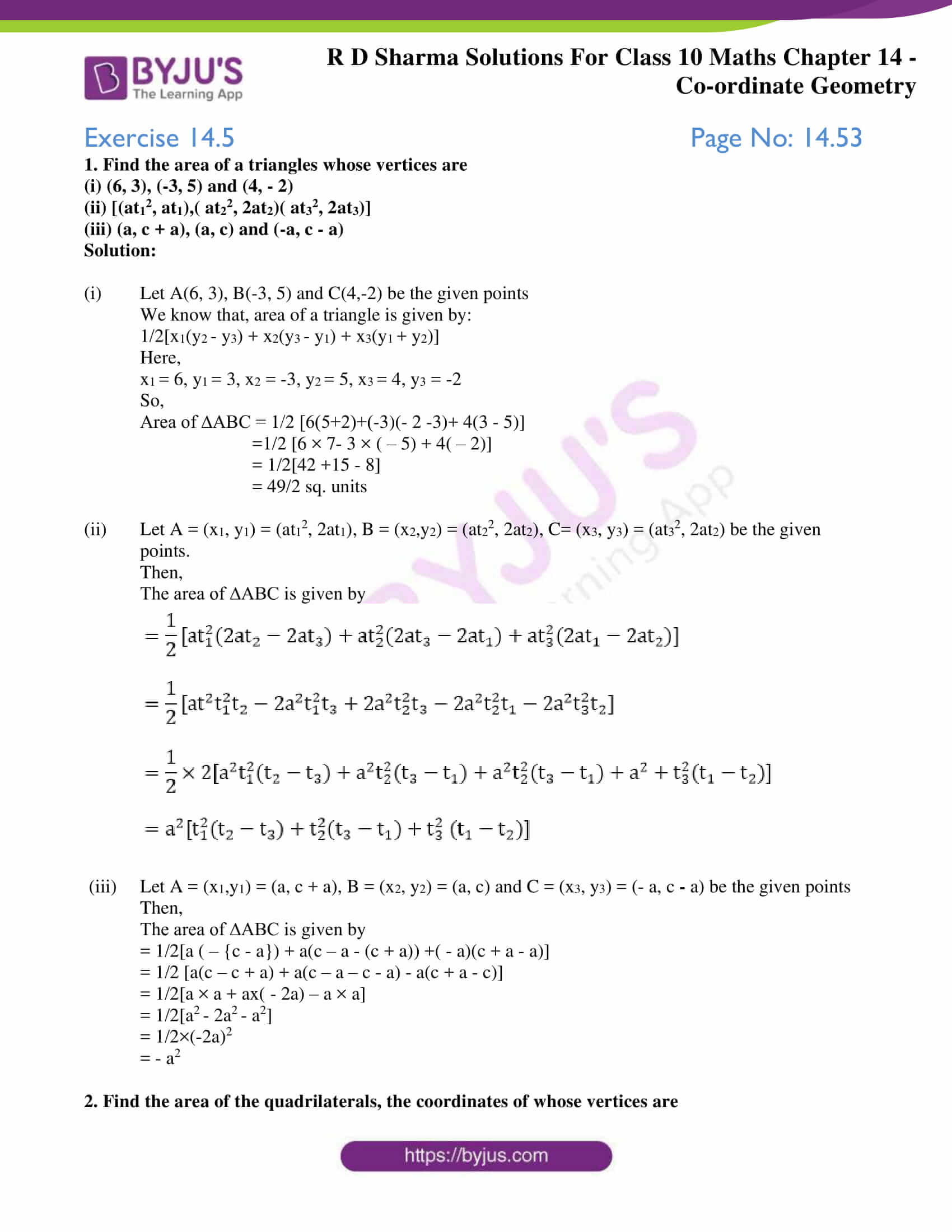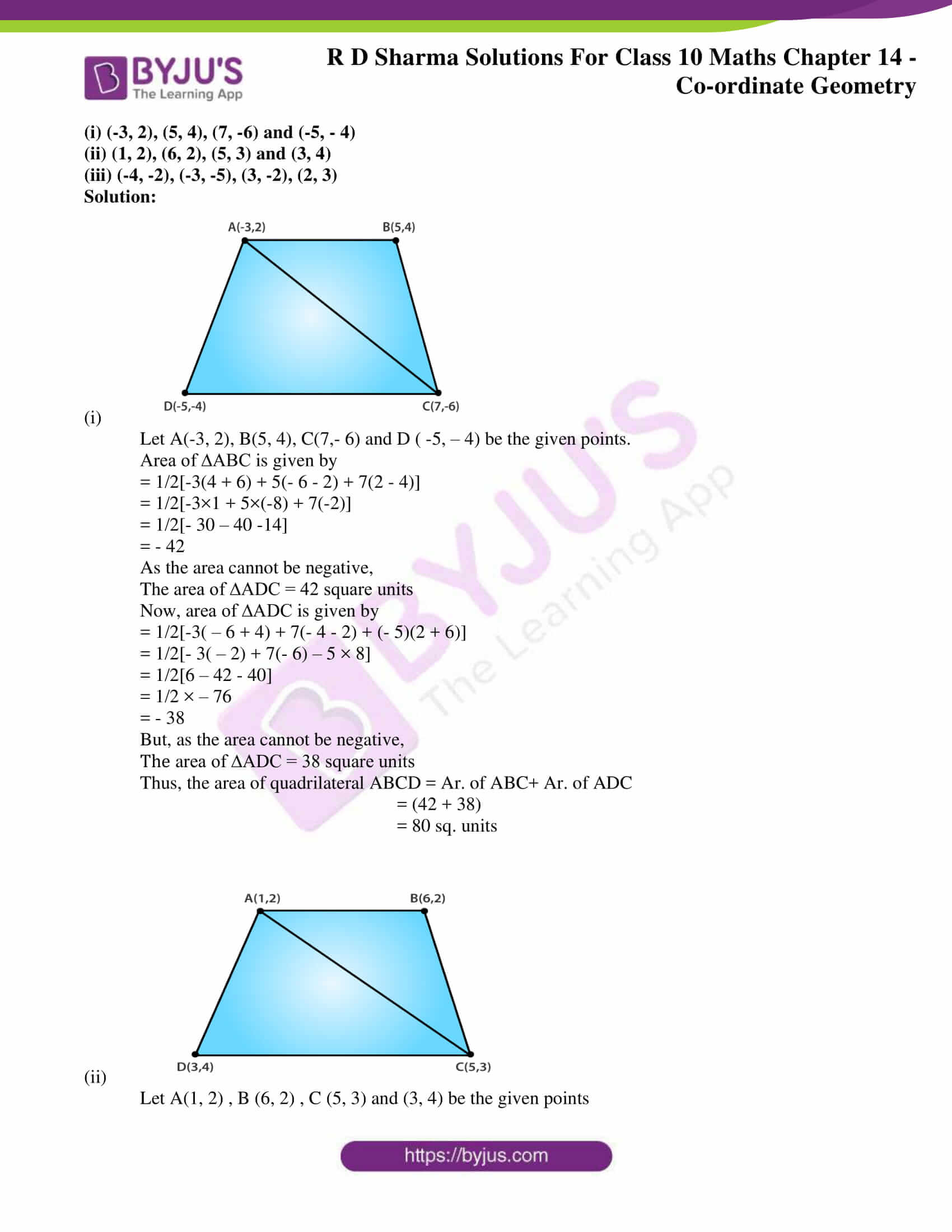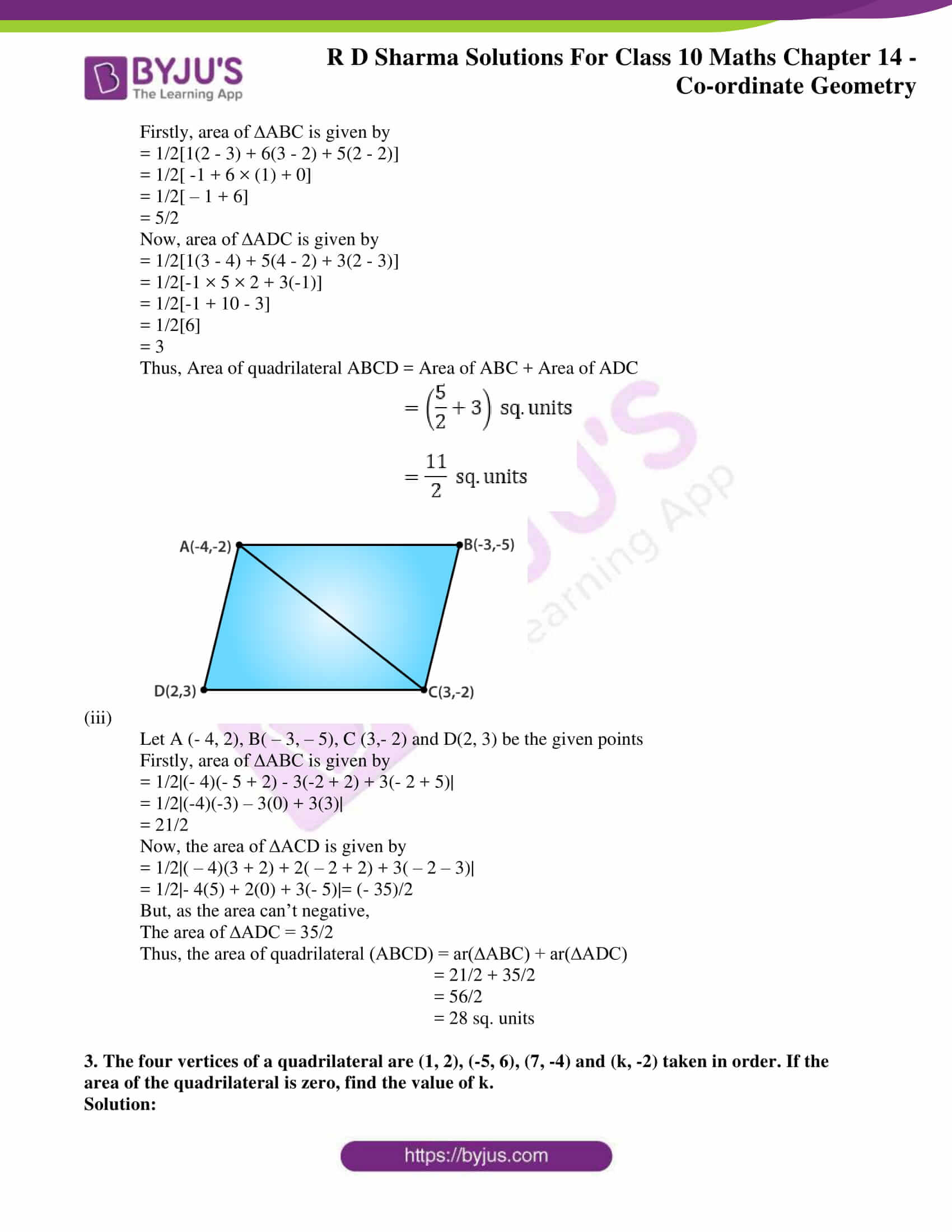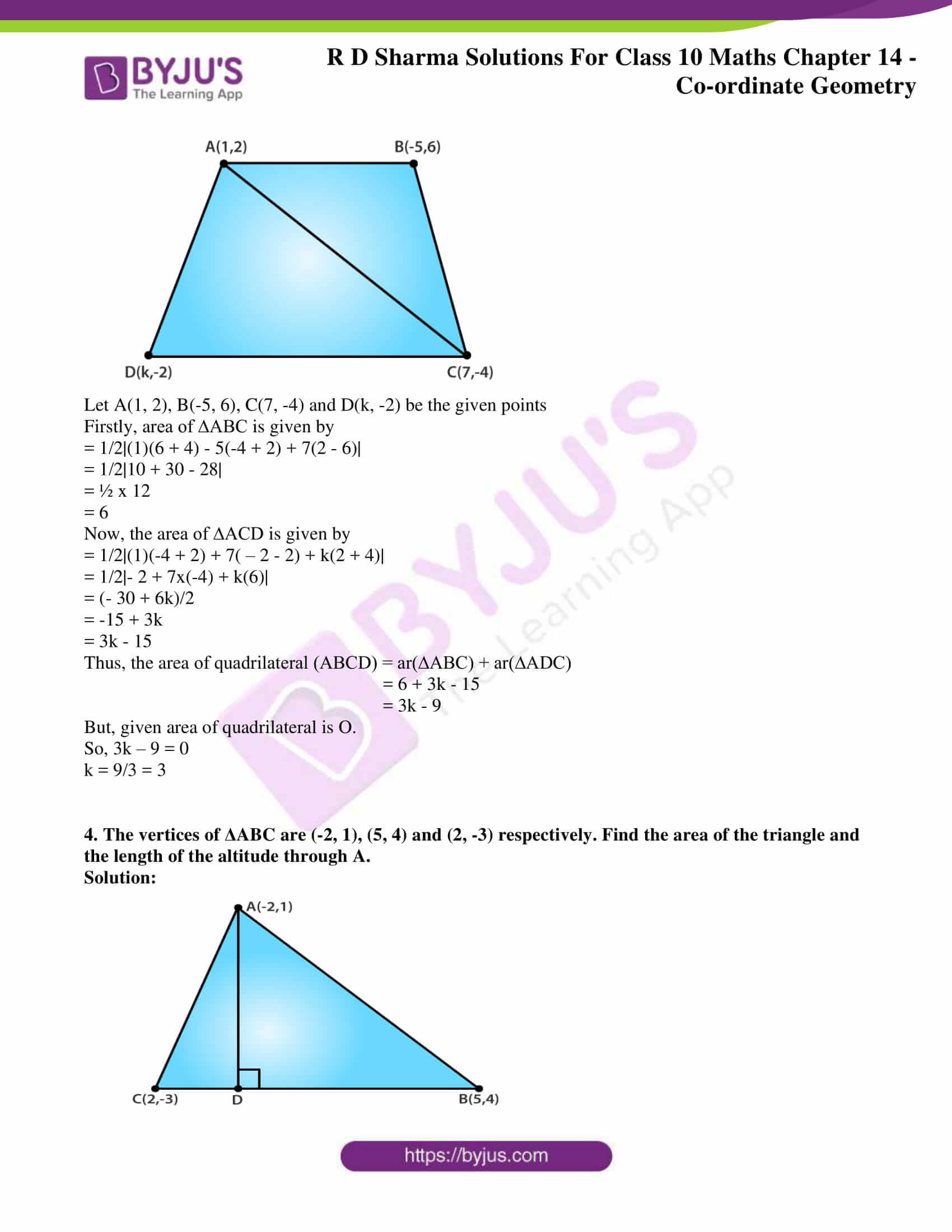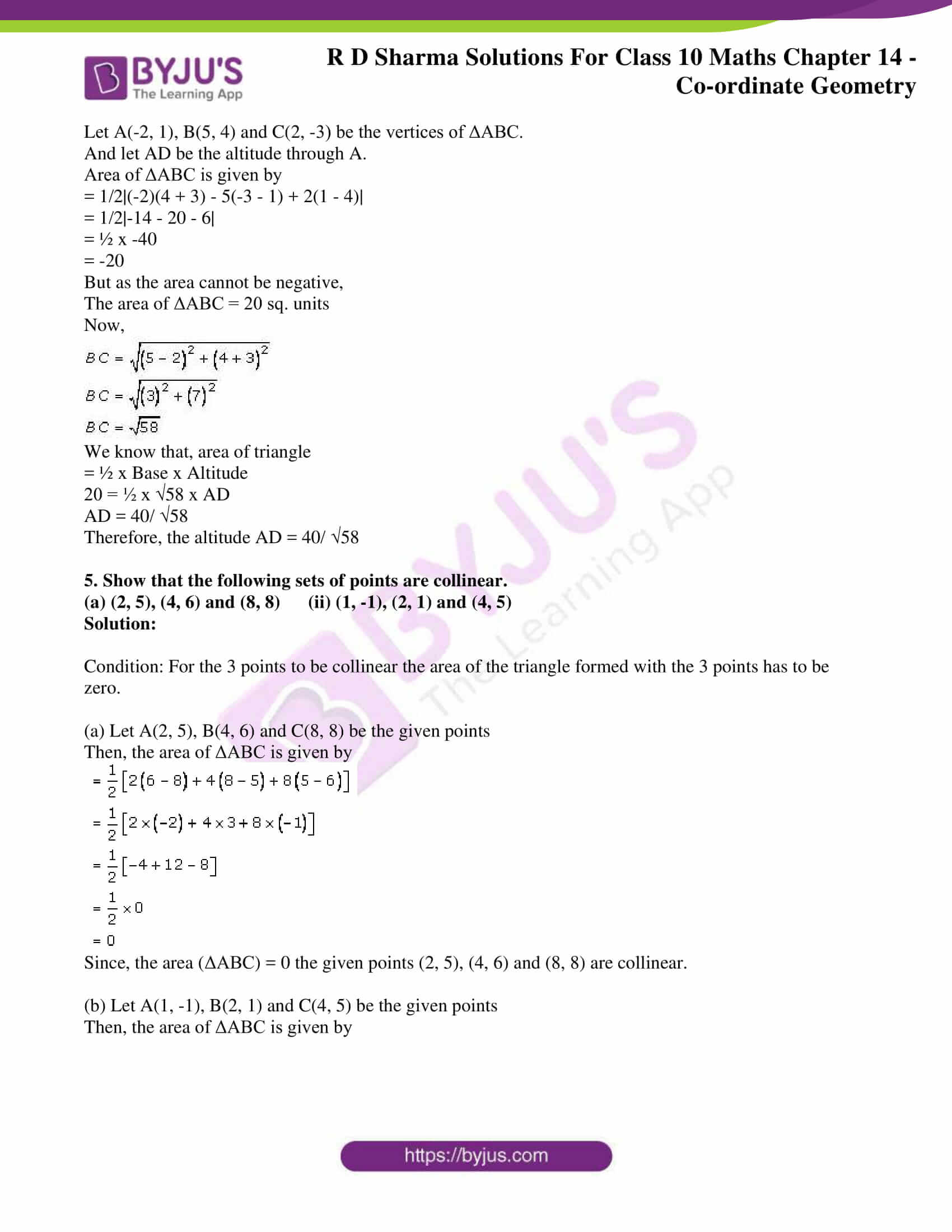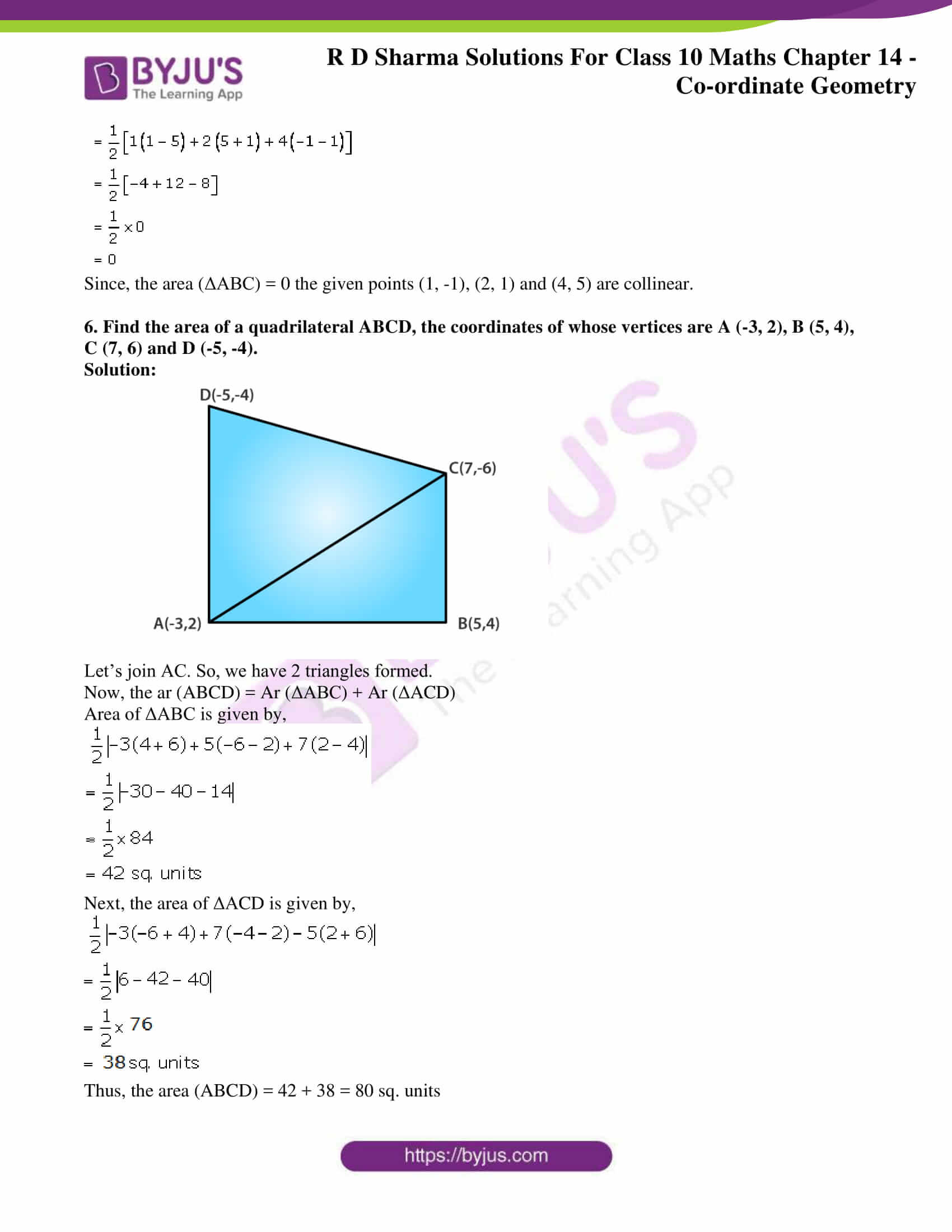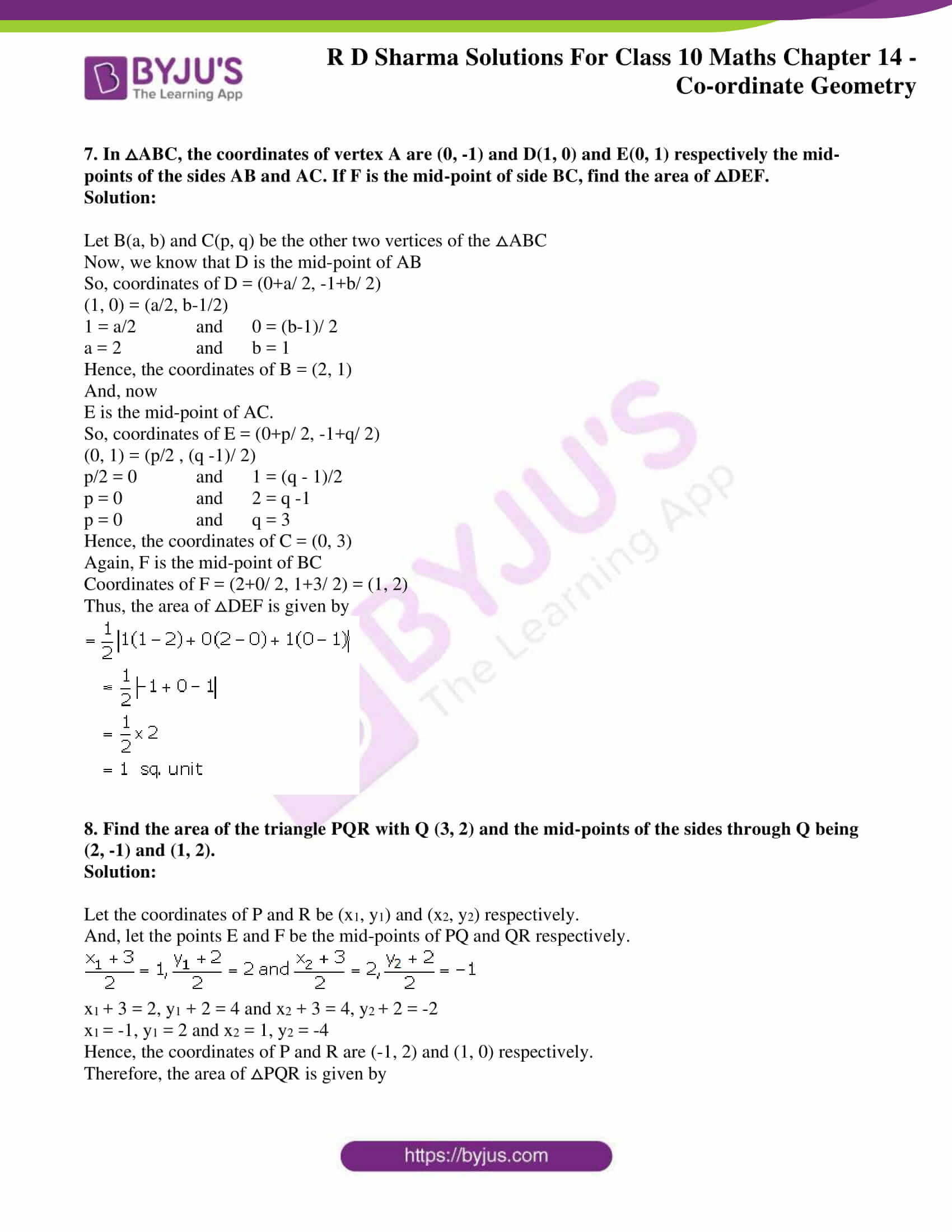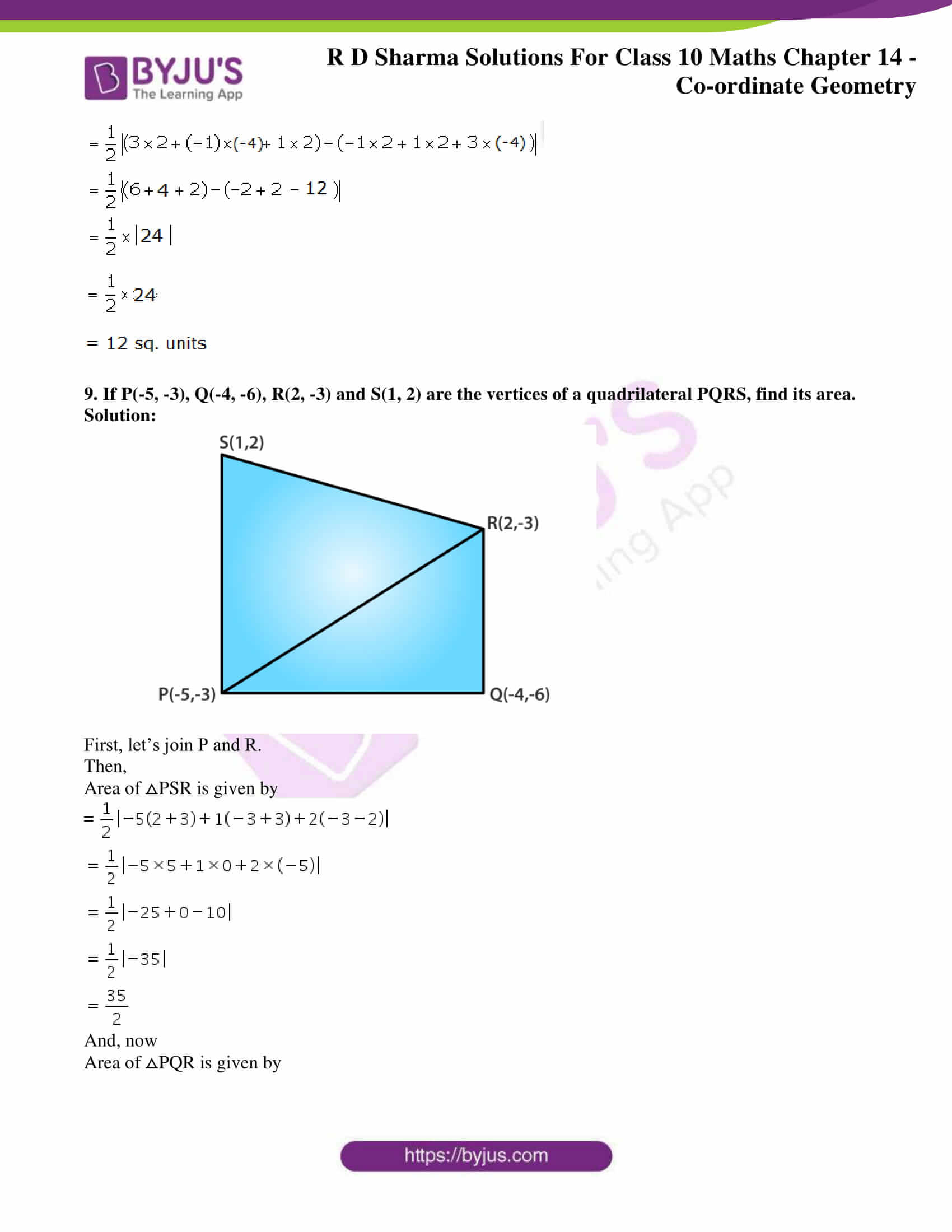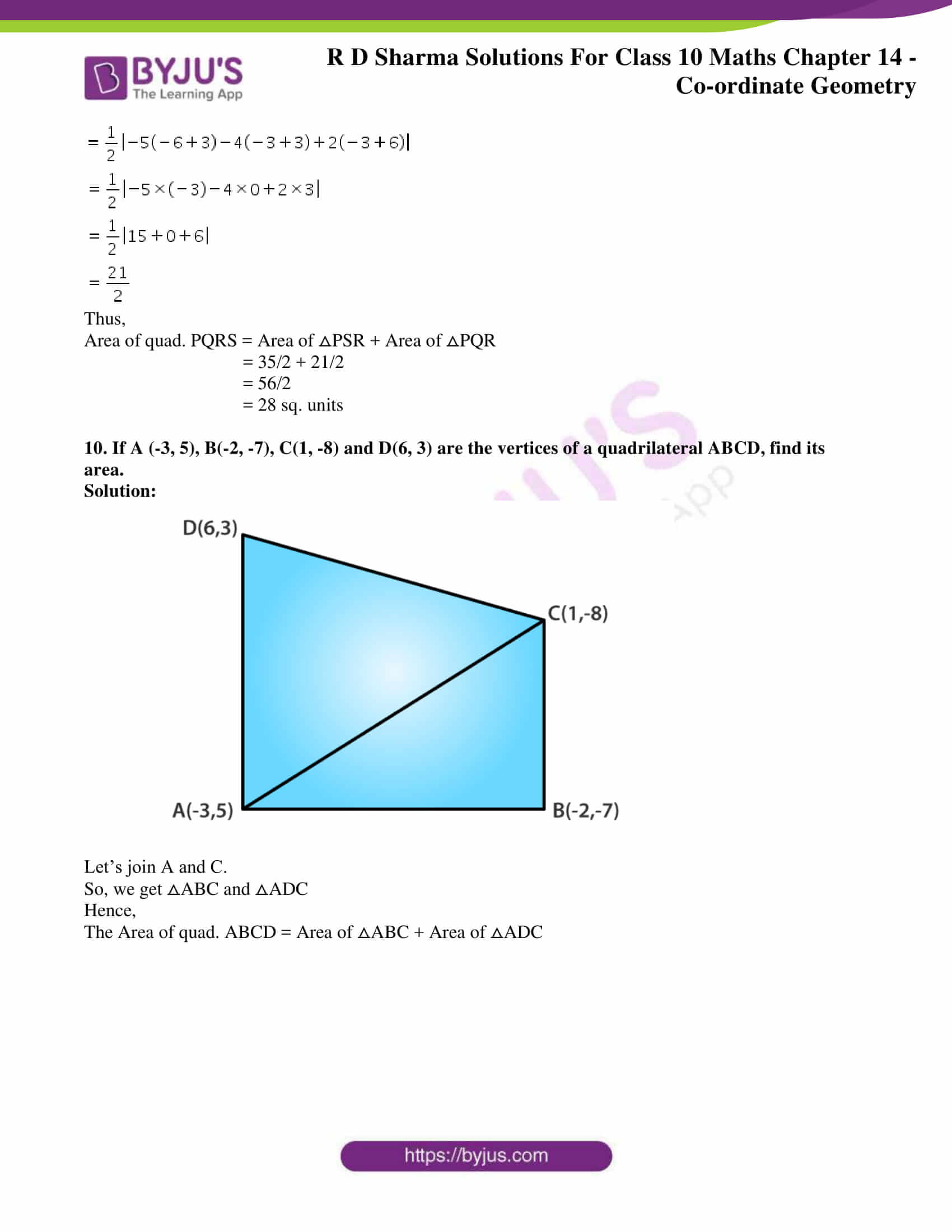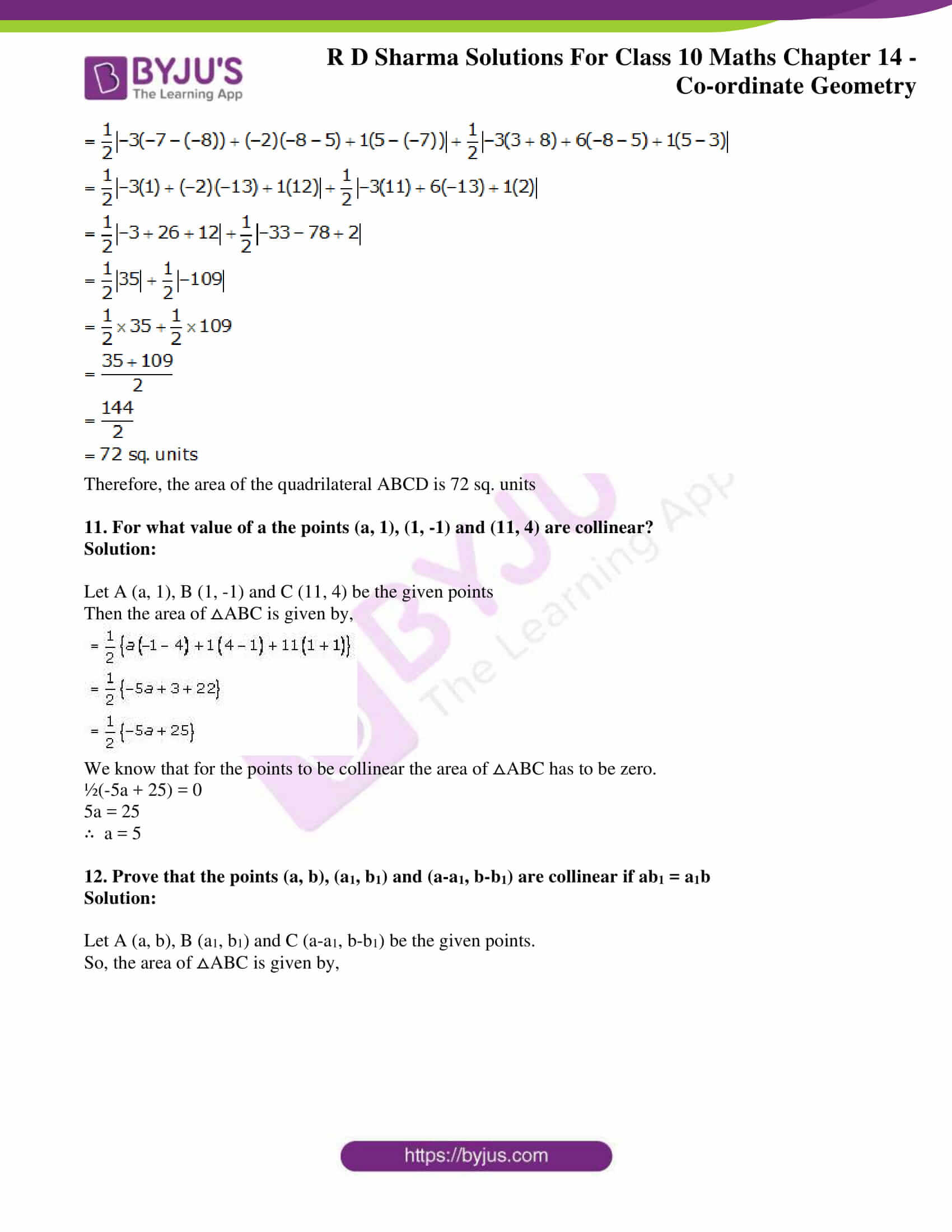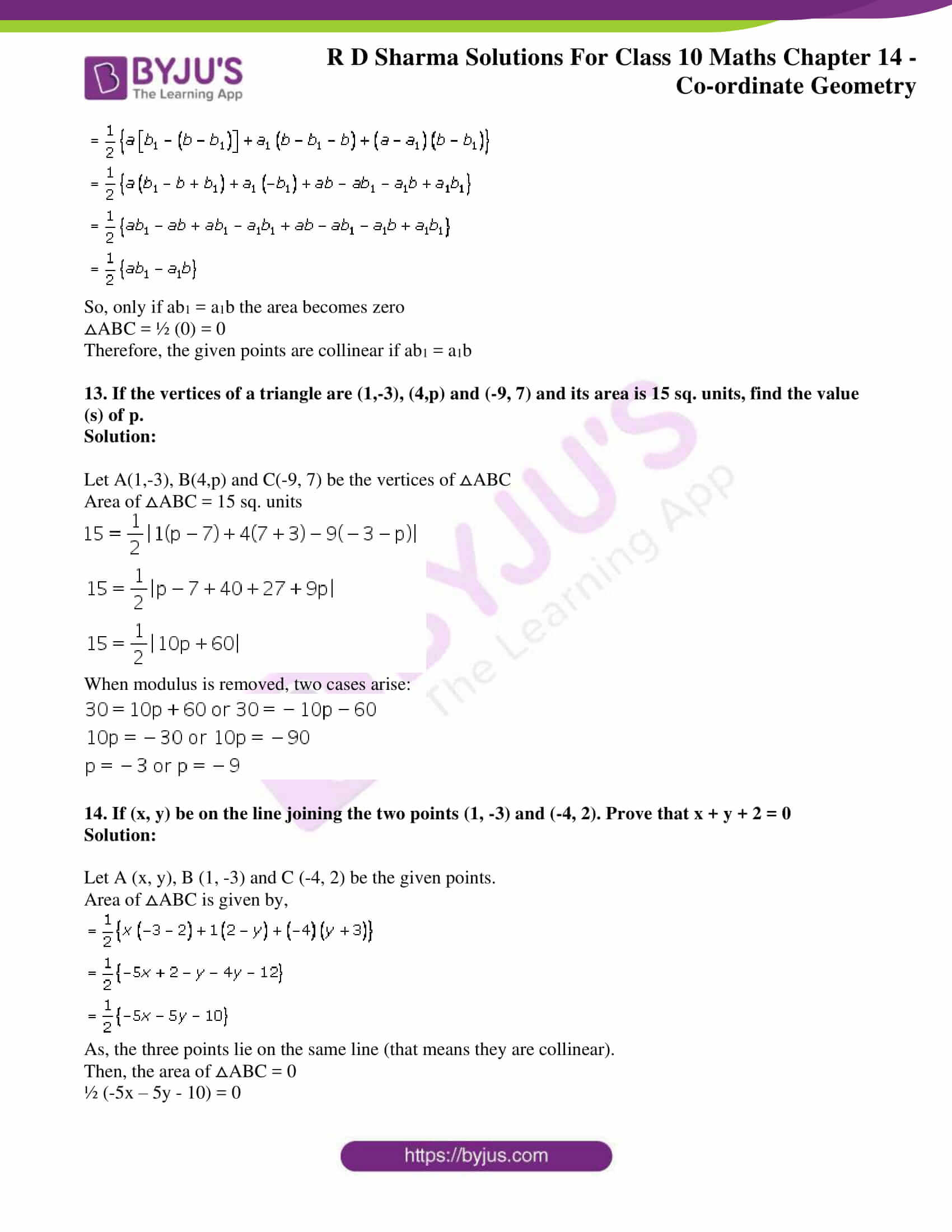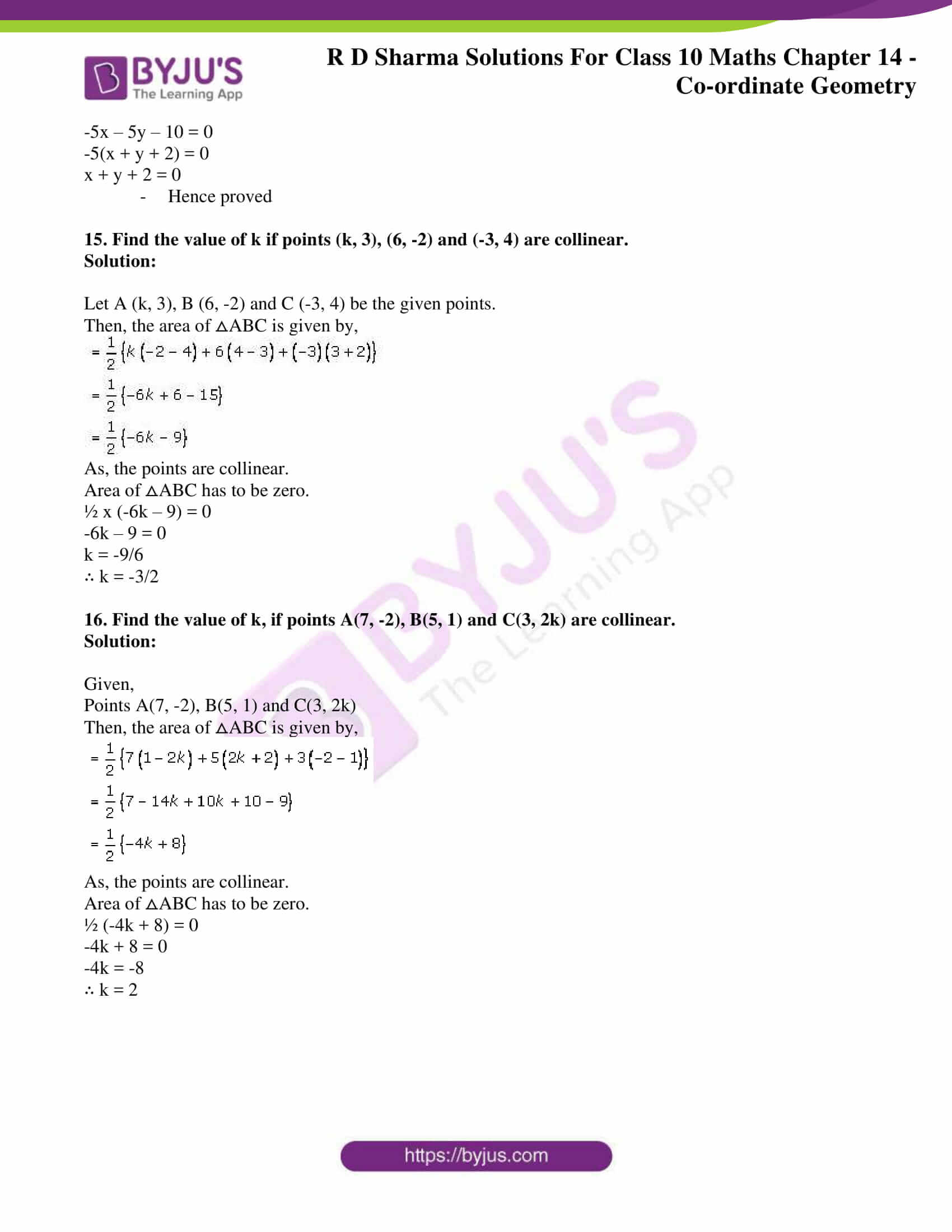### Access RD Sharma Solutions for Class 10 Maths Chapter 14 Co-ordinate Geometry Exercise 14.5

1. Find the area of a triangles whose vertices are

(i) (6, 3), (-3, 5) and (4, – 2)

(ii) [(at12, at1),( at22, 2at2)( at32, 2at3)]

(iii) (a, c + a), (a, c) and (-a, c – a)

Solution:

(i) Let A(6, 3), B(-3, 5) and C(4,-2) be the given points

We know that, area of a triangle is given by:

1/2[x1(y– y3) + x2(y– y1) + x3(y+ y2)]

Here,

x= 6, y= 3, x2 = -3, y= 5, x= 4, y3 = -2

So,

Area of ∆ABC = 1/2 [6(5+2)+(-3)(- 2 -3)+ 4(3 – 5)]

=1/2 [6 × 7- 3 × ( – 5) + 4( – 2)]

= 1/2[42 +15 – 8]

= 49/2 sq. units

(ii) Let A = (x1, y1) = (at12, 2at1), B = (x2,y2) = (at22, 2at2), C= (x3, y3) = (at32, 2at2) be the given points.

Then,

The area of ∆ABC is given by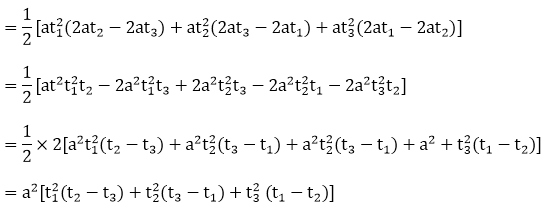(iii) Let A = (x1,y1) = (a, c + a), B = (x2, y2) = (a, c) and C = (x3, y3) = (- a, c – a) be the given points

Then,

The area of ∆ABC is given by

= 1/2[a ( – {c – a}) + a(c – a – (c + a)) +( – a)(c + a – a)]

= 1/2 [a(c – c + a) + a(c – a – c – a) – a(c + a – c)]

= 1/2[a × a + ax( – 2a) – a × a]

= 1/2[a– 2a– a2]

= 1/2×(-2a)2

= – a2

2. Find the area of the quadrilaterals, the coordinates of whose vertices are

(i) (-3, 2), (5, 4), (7, -6) and (-5, – 4)

(ii) (1, 2), (6, 2), (5, 3) and (3, 4)

(iii) (-4, -2), (-3, -5), (3, -2), (2, 3)

Solution:

(i)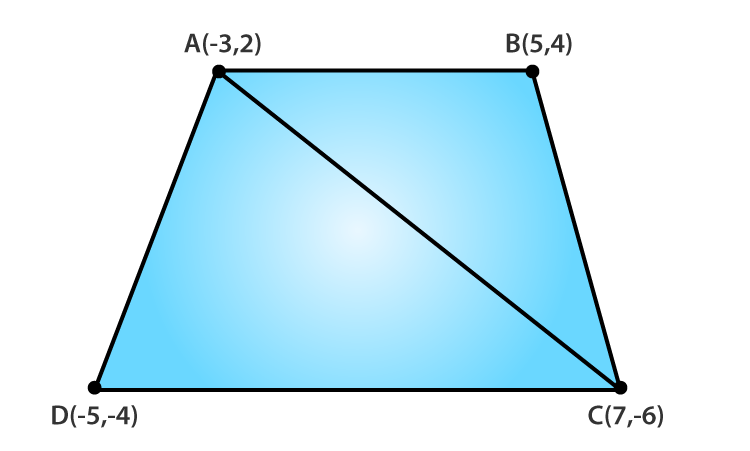Let A(-3, 2), B(5, 4), C(7,- 6) and D ( -5, – 4) be the given points.

Area of ∆ABC is given by

= 1/2[-3(4 + 6) + 5(- 6 – 2) + 7(2 – 4)]

= 1/2[-3×1 + 5×(-8) + 7(-2)]

= 1/2[- 30 – 40 -14]

= – 42

As the area cannot be negative,

The area of ∆ADC = 42 square units

Now, area of ∆ADC is given by

= 1/2[-3( – 6 + 4) + 7(- 4 – 2) + (- 5)(2 + 6)]

= 1/2[- 3( – 2) + 7(- 6) – 5 × 8]

= 1/2[6 – 42 – 40]

= 1/2 × – 76

= – 38

But, as the area cannot be negative,

The area of ∆ADC = 38 square units

Thus, the area of quadrilateral ABCD = Ar. of ABC+ Ar. of ADC

= (42 + 38)

= 80 sq. units

(ii)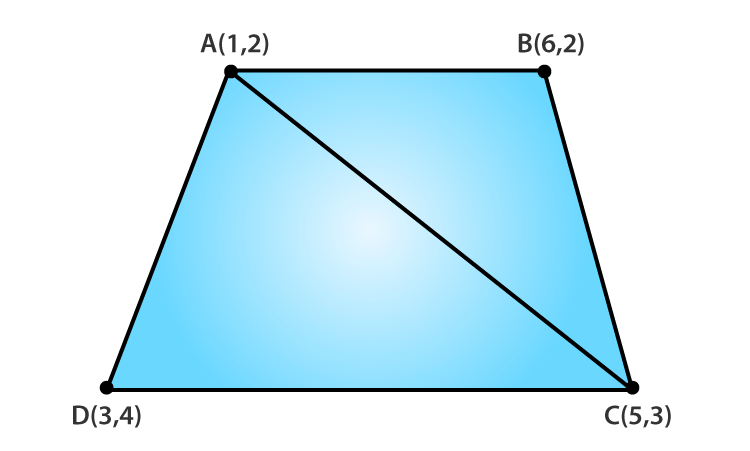Let A(1, 2) , B (6, 2) , C (5, 3) and (3, 4) be the given points

Firstly, area of ∆ABC is given by

= 1/2[1(2 – 3) + 6(3 – 2) + 5(2 – 2)]

= 1/2[ -1 + 6 × (1) + 0]

= 1/2[ – 1 + 6]

= 5/2

Now, area of ∆ADC is given by

= 1/2[1(3 – 4) + 5(4 – 2) + 3(2 – 3)]

= 1/2[-1 × 5 × 2 + 3(-1)]

= 1/2[-1 + 10 – 3]

= 1/2

= 3

Thus, Area of quadrilateral ABCD = Area of ABC + Area of ADC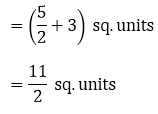(iii)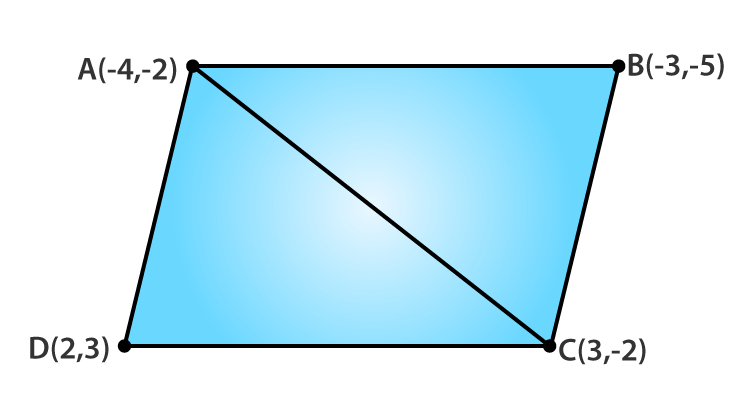Let A (- 4, 2), B( – 3, – 5), C (3,- 2) and D(2, 3) be the given points

Firstly, area of ∆ABC is given by

= 1/2|(- 4)(- 5 + 2) – 3(-2 + 2) + 3(- 2 + 5)|

= 1/2|(-4)(-3) – 3(0) + 3(3)|

= 21/2

Now, the area of ∆ACD is given by

= 1/2|( – 4)(3 + 2) + 2( – 2 + 2) + 3( – 2 – 3)|

= 1/2|- 4(5) + 2(0) + 3(- 5)|= (- 35)/2

But, as the area can’t negative,

The area of ∆ADC = 35/2

= 21/2 + 35/2

= 56/2

= 28 sq. units

3. The four vertices of a quadrilateral are (1, 2), (-5, 6), (7, -4) and (k, -2) taken in order. If the area of the quadrilateral is zero, find the value of k.

Solution: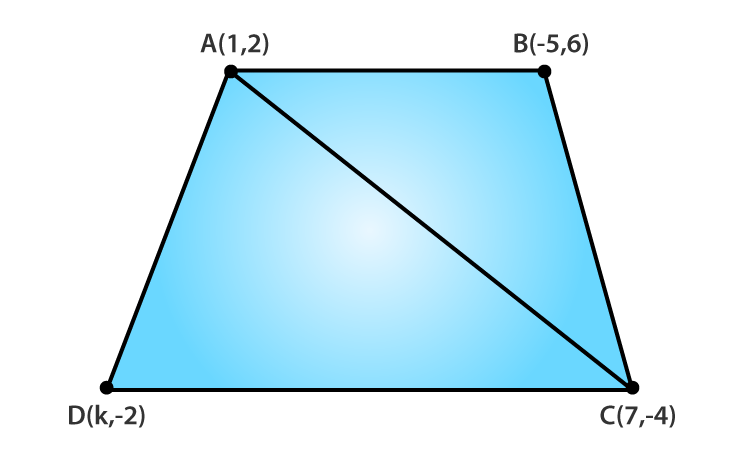Let A(1, 2), B(-5, 6), C(7, -4) and D(k, -2) be the given points

Firstly, area of ∆ABC is given by

= 1/2|(1)(6 + 4) – 5(-4 + 2) + 7(2 – 6)|

= 1/2|10 + 30 – 28|

= ½ x 12

= 6

Now, the area of ∆ACD is given by

= 1/2|(1)(-4 + 2) + 7( – 2 – 2) + k(2 + 4)|

= 1/2|- 2 + 7x(-4) + k(6)|

= (- 30 + 6k)/2

= -15 + 3k

= 3k – 15

= 6 + 3k – 15

= 3k – 9

But, given area of quadrilateral is O.

So, 3k – 9 = 0

k = 9/3 = 3

4. The vertices of ΔABC are (-2, 1), (5, 4) and (2, -3) respectively. Find the area of the triangle and the length of the altitude through A.

Solution: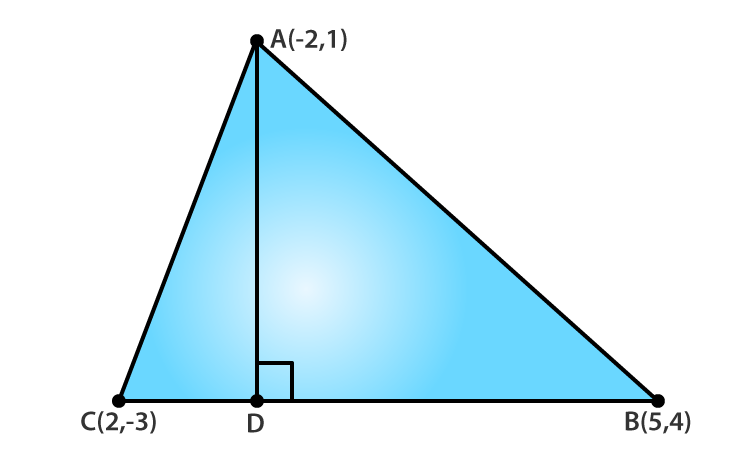Let A(-2, 1), B(5, 4) and C(2, -3) be the vertices of ΔABC.

And let AD be the altitude through A.

Area of ΔABC is given by

= 1/2|(-2)(4 + 3) – 5(-3 – 1) + 2(1 – 4)|

= 1/2|-14 – 20 – 6|

= ½ x -40

= -20

But as the area cannot be negative,

The area of ΔABC = 20 sq. units

Now,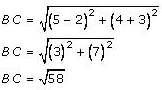We know that, area of triangle

= ½ x Base x Altitude

20 = ½ x √58 x AD

Therefore, the altitude AD = 40/ √58

5. Show that the following sets of points are collinear.

(a) (2, 5), (4, 6) and (8, 8) (ii) (1, -1), (2, 1) and (4, 5)

Solution:

Condition: For the 3 points to be collinear the area of the triangle formed with the 3 points has to be zero.

(a) Let A(2, 5), B(4, 6) and C(8, 8) be the given points

Then, the area of ΔABC is given by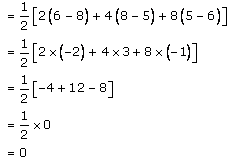Since, the area (ΔABC) = 0 the given points (2, 5), (4, 6) and (8, 8) are collinear.

(b) Let A(1, -1), B(2, 1) and C(4, 5) be the given points

Then, the area of ΔABC is given by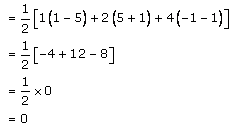Since, the area (ΔABC) = 0 the given points (1, -1), (2, 1) and (4, 5) are collinear.

6. Find the area of a quadrilateral ABCD, the coordinates of whose vertices are A (-3, 2), B (5, 4), C (7, 6) and D (-5, -4).

Solution: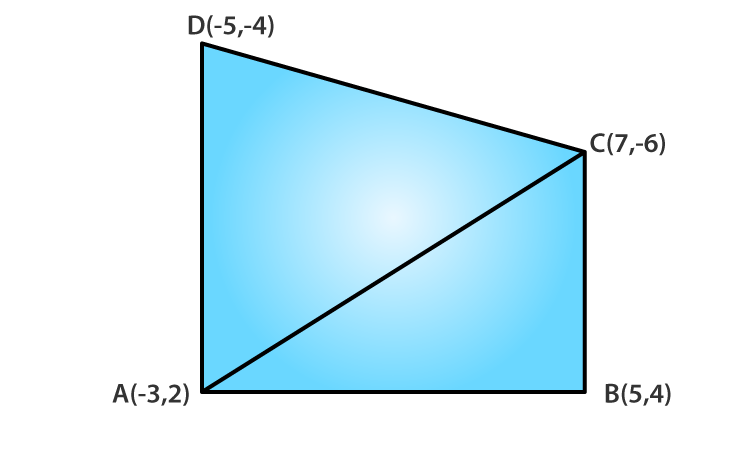Let’s join AC. So, we have 2 triangles formed.

Now, the ar (ABCD) = Ar (ΔABC) + Ar (ΔACD)

Area of ΔABC is given by,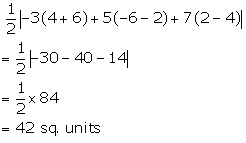Next, the area of ΔACD is given by,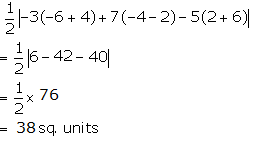Thus, the area (ABCD) = 42 + 38 = 80 sq. units

7. In ⧍ABC, the coordinates of vertex A are (0, -1) and D(1, 0) and E(0, 1) respectively the mid-points of the sides AB and AC. If F is the mid-point of side BC, find the area of ⧍DEF.

Solution:

Let B(a, b) and C(p, q) be the other two vertices of the ⧍ABC

Now, we know that D is the mid-point of AB

So, coordinates of D = (0+a/ 2, -1+b/ 2)

(1, 0) = (a/2, b-1/2)

1 = a/2 and 0 = (b-1)/ 2

a = 2 and b = 1

Hence, the coordinates of B = (2, 1)

And, now

E is the mid-point of AC.

So, coordinates of E = (0+p/ 2, -1+q/ 2)

(0, 1) = (p/2 , (q -1)/ 2)

p/2 = 0 and 1 = (q – 1)/2

p = 0 and 2 = q -1

p = 0 and q = 3

Hence, the coordinates of C = (0, 3)

Again, F is the mid-point of BC

Coordinates of F = (2+0/ 2, 1+3/ 2) = (1, 2)

Thus, the area of ⧍DEF is given by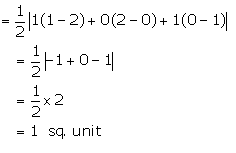8. Find the area of the triangle PQR with Q (3, 2) and the mid-points of the sides through Q being (2, -1) and (1, 2).

Solution:

Let the coordinates of P and R be (x1, y1) and (x2, y2) respectively.

And, let the points E and F be the mid-points of PQ and QR respectively.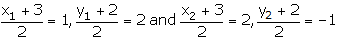x1 + 3 = 2, y1 + 2 = 4 and x2 + 3 = 4, y2 + 2 = -2

x1 = -1, y1 = 2 and x2 = 1, y2 = -4

Hence, the coordinates of P and R are (-1, 2) and (1, 0) respectively.

Therefore, the area of ⧍PQR is given by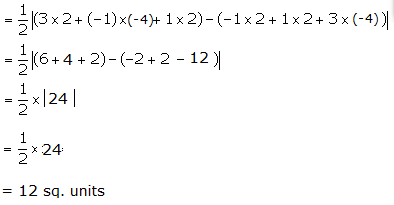9. If P(-5, -3), Q(-4, -6), R(2, -3) and S(1, 2) are the vertices of a quadrilateral PQRS, find its area.

Solution: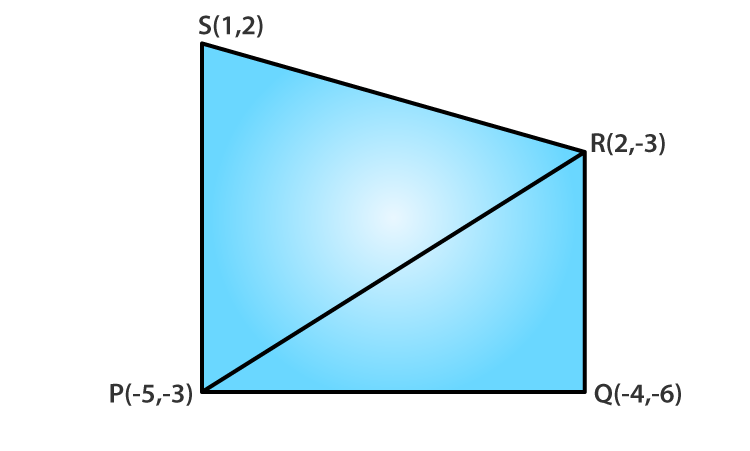First, let’s join P and R.

Then,

Area of ⧍PSR is given by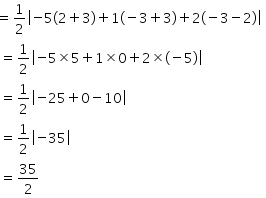And, now

Area of ⧍PQR is given by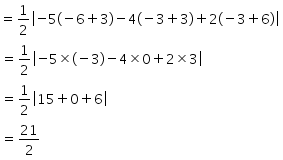Thus,

Area of quad. PQRS = Area of ⧍PSR + Area of ⧍PQR

= 35/2 + 21/2

= 56/2

= 28 sq. units

10. If A (-3, 5), B(-2, -7), C(1, -8) and D(6, 3) are the vertices of a quadrilateral ABCD, find its area.

Solution: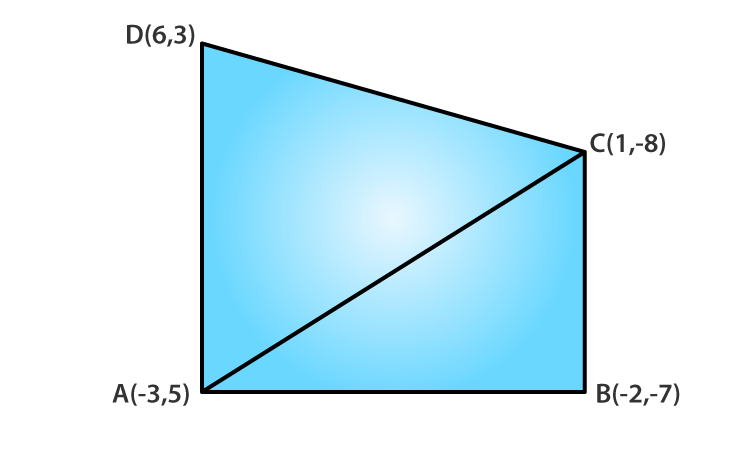Let’s join A and C.

So, we get ⧍ABC and ⧍ADC

Hence,

The Area of quad. ABCD = Area of ⧍ABC + Area of ⧍ADC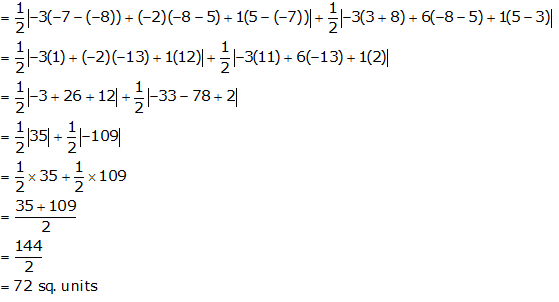Therefore, the area of the quadrilateral ABCD is 72 sq. units

11. For what value of a the points (a, 1), (1, -1) and (11, 4) are collinear?

Solution:

Let A (a, 1), B (1, -1) and C (11, 4) be the given points

Then the area of ⧍ABC is given by,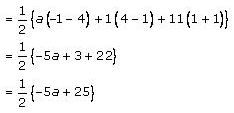We know that for the points to be collinear the area of ⧍ABC has to be zero.

½(-5a + 25) = 0

5a = 25

∴ a = 5

12. Prove that the points (a, b), (a1, b1) and (a-a1, b-b1) are collinear if ab1 = a1b

Solution:

Let A (a, b), B (a1, b1) and C (a-a1, b-b1) be the given points.

So, the area of ⧍ABC is given by,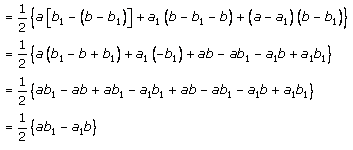So, only if ab1 = a1b the area becomes zero

⧍ABC = ½ (0) = 0

Therefore, the given points are collinear if ab1 = a1b

13. If the vertices of a triangle are (1,-3), (4,p) and (-9, 7) and its area is 15 sq. units, find the value (s) of p.

Solution:

Let A(1,-3), B(4,p) and C(-9, 7) be the vertices of ⧍ABC

Area of ⧍ABC = 15 sq. units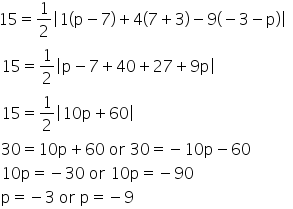When modulus is removed, two cases arise:14. If (x, y) be on the line joining the two points (1, -3) and (-4, 2). Prove that x + y + 2 = 0

Solution:

Let A (x, y), B (1, -3) and C (-4, 2) be the given points.

Area of ⧍ABC is given by,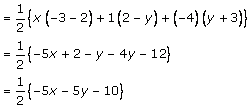As, the three points lie on the same line (that means they are collinear).

Then, the area of ⧍ABC = 0

½ (-5x – 5y – 10) = 0

-5x – 5y – 10 = 0

-5(x + y + 2) = 0

x + y + 2 = 0

• Hence proved

15. Find the value of k if points (k, 3), (6, -2) and (-3, 4) are collinear.

Solution:

Let A (k, 3), B (6, -2) and C (-3, 4) be the given points.

Then, the area of ⧍ABC is given by,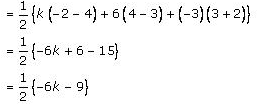As, the points are collinear.

Area of ⧍ABC has to be zero.

½ x (-6k – 9) = 0

-6k – 9 = 0

k = -9/6

∴ k = -3/2

16. Find the value of k, if points A(7, -2), B(5, 1) and C(3, 2k) are collinear.

Solution:

Given,

Points A(7, -2), B(5, 1) and C(3, 2k)

Then, the area of ⧍ABC is given by,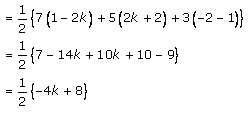As, the points are collinear.

Area of ⧍ABC has to be zero.

½ (-4k + 8) = 0

-4k + 8 = 0

-4k = -8

∴ k = 2